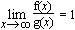# asymptotically equal

One way of saying that two functions f(x) and g(x) are about the same size is to say that they are asymptotically equal: Two functions f(x) and g(x)are asymptotically equal (as x approaches infinity) if the following limit holds:This is often denoted f(x)~ g(x).

For example: 3x4+2x+7 ~ 3x4, x+sin x ~ x, and the prime number theorem states that pi(x) ~ x/ln x.

If f(x)~g(x), then f is O(g) and g is O(f), but the converse is not true. Equivalently, if f(x)~ g(x), then g has the same order of magnitude as f (and again the converse is false). Finally, if f(x)~ g(x), then f(x)- g(x) is o(g(x)).